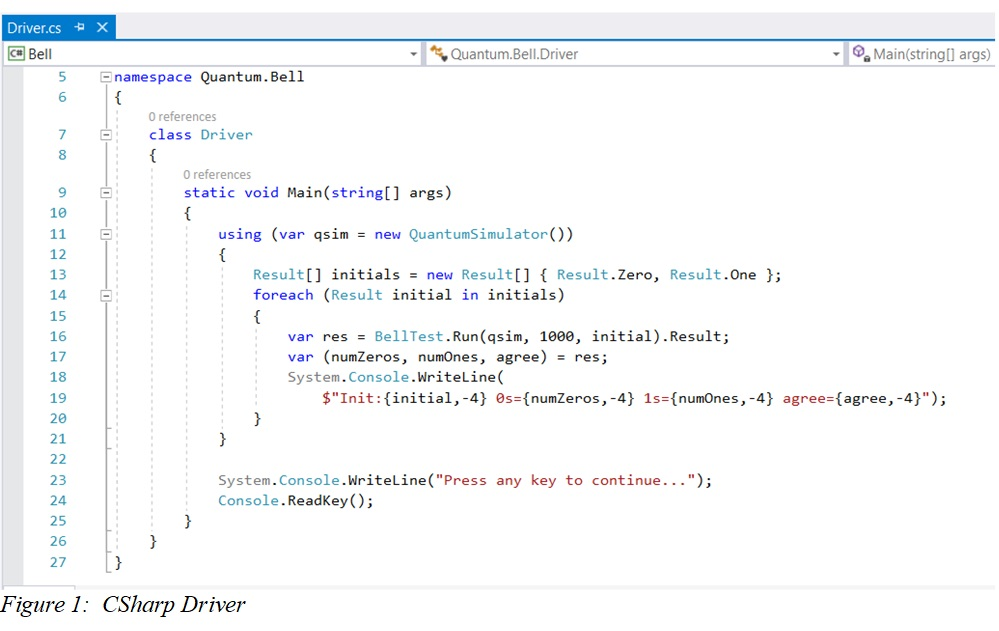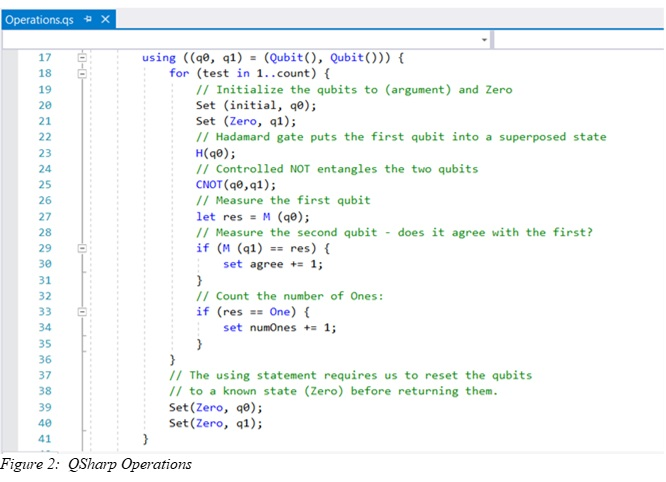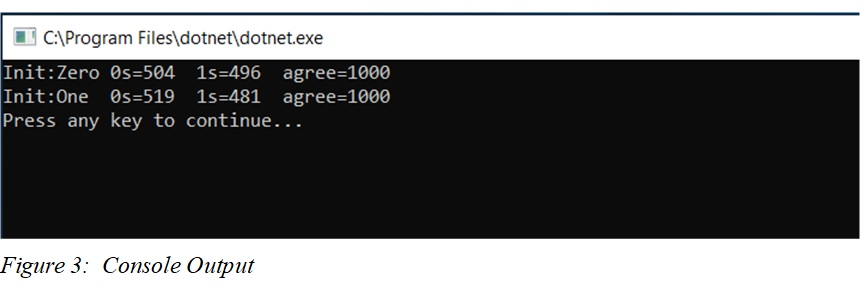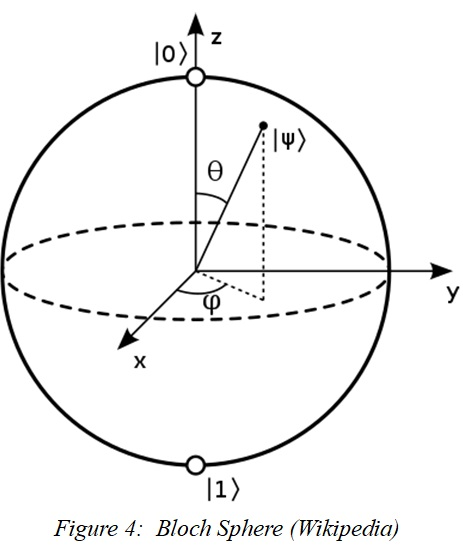# Quantum Computing at the Kitchen Table

## Introduction

Recently, Google published a claim that they got a quantum computer with 53 superconducting qubits to do a calculation in 200 seconds that would have taken a classical computer 10,000 years to complete.  They don’t claim it was a useful calculation (more like a good random number), but just getting an actual quantum computer to do anything like this is a milestone.

IBM immediately fired back “the same task can be performed on a classical system in 2.5 days.”  This is a quibble about the architecture of a theoretical classical computer but appears to concede the main point.  A quantum computer has been shown to have an edge on a classical one.  Of course, IBM is building its own quantum computer, as is Microsoft.

Microsoft lets you download a software development kit you can use to write and run your own quantum programs.  This is a simulator, of course, but it isn’t a toy.  It’s the infrastructure you would need to talk to a real quantum computer and make practical use of it.

You download a Visual Studio extension QsharpVSIX.vsix and set it up.  Then you write a C# driver program that invokes quantum operations, retrieves the results, and displays them for you.  That part is all classical.

Next, you write the quantum program itself in a new language called Q#.  This is what runs in the simulator.  There are a few different simulators.  If you instantiate a QuantumSimulator, you get a full state vector simulation of up to 30 or so qubits, though you’re probably looking for a bigger computer if you really want to use that many.  There is a ResourcesEstimator target machine that doesn’t actually do the calculation, but tells you how many resources your program is calling for, up to few thousand qubits.  Finally, there is a ToffoliSimulator that only does the simplest quantum gates that can be efficiently simulated on a classical computer but can handle millions of qubits.

There is an elegant walkthrough for how to use the full QuantumSimulator to set up an entangled pair of qubits (a Bell pair) and then carry out a classical measurement on them.

## “Einstein was Wrong”

Everybody always likes to say things like “Einstein was Wrong.”  Of course, because he worked on a lot of new ideas, he was sometimes wrong.  And if someone else got it right, he acknowledged it.  That’s how science works.

Einstein was skeptical of quantum mechanics.  In 1935, Einstein, Podolsky and Rosen (EPR) came up with an argument that was intended to show that quantum mechanics couldn’t be a complete description of reality.  One version of this thought experiment (different from their original argument) starts with a spin zero state which decays into two photons heading off in opposite directions.  A photon has spin one  –  either +1 or -1  –  and the two spins are entangled in the sense that they have to add up to zero because angular momentum is conserved.  Each photon starts off in a superposition of the +1 and -1 states.

You let them head off in opposite directions, and then you perform a classical measurement on each spin.  Crucially, you do this when they are so far apart that no one could get a light speed message from one place of measurement to the other.  The measured spins must add up to zero.  This means that when the first measurement comes out as +1 (for example), the other photon instantly  –  faster than light speed  –  has to go into the -1 state.  That’s what quantum mechanics predicts.

This doesn’t actually violate causality or special relativity, since no information can be sent this way (neither side knows what to expect!), but it sure looks like “spooky action at a distance”.  Einstein anticipated that this spooky action wouldn’t happen because reality should be “local”.  Unfortunately for Einstein, the universe really does work that way.  We’ve done the experiment.

Later, in 1964, John Bell provided a way to check that there is no “hidden variable” somewhere that already knows the answer.  The result really does get decided when the first measurement is performed.  Today, this experimental setup is known either as an EPR pair or as a Bell pair.  We can run a simulated version of this experiment in Q#, and if we can get our hands on a quantum computer, the same program would run the experiment for real.

## Bell Test

The walkthrough begins by showing us how to set up a C# driver program:Here, the computational basis is 0 and 1 instead of +1 and -1, and we’re going to set up the entanglement such that the two qubits are constrained to agree with each other (both zero or both one) instead of being opposite.  But the idea is the same.

We are going to start with a single qubit initialized to either zero or one, and we’re going to run 1000 simulations each way.  We’re expecting back a result that tells us how many times the first qubit was measured to be a Zero, how many times it was measured to be a One, and how many times the second qubit agreed with the first.

Here are the instructions for our simulated quantum computer:And here are the results of a trial run:The first qubit was rotated into a superposed state, so it was measured to be a One about half the time, regardless of its initialized value.  The second qubit was also in a superposed state, but it was entangled with the first qubit in such a way that its measurement always comes out the same as the first.  You can perform the measurements in the opposite order, and you get the same outcome.

This demonstration used a Hadamard gate H(q0) to put the first qubit into a superposed state, and it also used a Controlled NOT gate CNOT(q0,q1) to entangle the two qubits.  There are other gates to do flips and rotations, but they can all be assembled out of H() and CNOT() gates, in the same way that all classical gates can be built out of NAND gates (and fanouts).

This doesn’t, however, demonstrate a quantum speedup.  To do that, you have to get the entangled states to perform some useful calculation in parallel, and then get the states to interfere with each other in such a way that your classical measurement of their final state tells you something interesting about that calculation.  I have not yet stepped through Grover’s quantum search algorithm and Shor’s integer factorization algorithm, but example code for these algorithms is included with the software development kit.

## What’s So Exciting about Quantum Computing, Anyway?

There are two rather different perspectives on quantum computing, the mathematician’s perspective and the programmer’s perspective.  They are interested in somewhat different things, roughly corresponding to theoretical and practical possibilities.

I have some interest in both sides.  I passed both semesters of graduate quantum mechanics, but did not take field theory, and went on to twenty years of programming for business.  I’ve been looking through the standard textbook Quantum Computing and Quantum Information by Michael Nielsen and Isaac Chuang.  I tend to be a bit skeptical of math that lacks practical applications, but I’m also looking for opportunities.

The mathematician is coming from the twentieth century’s breakthrough insights into the fundamental nature of algorithms and what is computable.  You’ve probably heard about Alan Turing’s work on breaking the German Enigma code during World War II, and the Turing Test for artificial intelligence (“can it fool me into thinking it’s a human?”).  But that’s not what Turing is famous for.  What he’s famous for is the 1935 insight that a simple conceptual model of a computer – this is before there were real electronic computers – can perform a series of operations that cover everything computable.  It defines what an algorithm is, and what problems it can solve, in principle, on any computer.

Now, a Turing model computer is just conceptual.  It’s an infinitely long indestructible tape with an indestructible read/write head that can move the tape and scribble on it.  This is not a practical computer  –  because it’s not efficient from a programmer’s point of view.  But it is useful for telling you what you can do with a classical computer.

A quantum computer doesn’t break this picture.  It can’t compute anything that isn’t computable on a classical computer.  It operates on the same set of algorithms.  However, there are algorithms which can be computed more efficiently on a quantum computer than on a classical computer.  That’s what the excitement is about.

In this context, “efficient” means something specific to a mathematician.  The mathematician may consider a problem “efficiently computable” even when it is completely impractical from the programmer’s point of view, and the mathematician may consider a problem “not efficiently computable” (for a sufficiently large version of the problem) even though the programmer implements practical solutions (for relatively small versions of the problem) every day.

What is this “size” of the problem, that bears so directly on whether it can be “efficiently computed”?

We can count the pieces in various problems, and then ask how many steps we have to perform to solve that problem.  For example, we can count the number of cities a travelling salesman has to visit, the number of binary digits in a number we have to break down into its prime factors, or the number of records in a database table that we have to search for a record we want.  The number of pieces in the problem is an integer:  n.

The mathematician sees a fundamental distinction between problems where the number of steps to a solution scales like to some power, like n, or n2, or even n100  –  and problems where appears in the exponent, like 2n.  The first kind of problem is known to be solvable in “polynomial time” and can be efficiently computed on a classical Turing machine.  The second kind of problem quickly involves an astronomical number of steps and, as far as we know, cannot be efficiently computed on a classical Turing machine.

These two kinds of problems are described as complexity classes “P” (for “polynomial”) and “NP”.  An outstanding problem in mathematics is whether “NP” problems – like the travelling salesman’s problem of minimizing the distance travelled to visit all cities – might not be solvable in polynomial time after all.  That is believed to be impossible, but no one has proved that it is impossible.

Quantum computers can, in theory, solve some of these problems “efficiently”.  No one has yet come up with a way to do this for the travelling salesman problem.  However, we know that a sufficiently large quantum computer could use Shor’s integer factorization to break RSA public keys and decrypt all of our HTTPS traffic.  We would then have to go back to using private keys.

However, even as quantum computing takes with one hand, it gives with the other.  Quantum key distribution makes it possible to share a private key even over a public channel, since an eavesdropper can’t read the key without destroying it.  And quantum key distribution has been demonstrated in practice.

## Hardware and Present Limitations

You can in principle build a quantum computer using any two-level quantum state to represent a qubit.  There are plenty of these in nature.  For example, an electron spin is +1/2 or -1/2, while a photon spin is +1 or -1 (no zero), and you can even get at the nuclear spin inside atoms using an NMR machine.  But you’re not limited to spins.  You can also use charge, current, and magnetic states.  It generally helps to cool things down to superconducting temperatures to minimize thermal noise and stretch out the decoherence time.  (Decoherence time is how long your qubit stays in its original state before it undergoes an unwanted classical measurement at the hands of its environment.)

A qubit that uses spins is helpful for visualization, since you can imagine the spin axis pointing to any spot on a Bloch sphere, and those two angles can be mapped to the two complex coefficients that define the state.This gives you a feeling for the kind of information that is present in the qubit and can be entangled with other qubits.  You can’t read out all of that information, of course.  When you finally carry out a classical measurement on the qubit you will just get a 1 or a 0, each with probability equal to the square of its coefficient.  That’s what you have to go on when you’re trying to figure out the result of a more complicated interaction among many qubits.

Thinking of it as a spin arrow also helps me visualize a superposed state.  For example, when you prepare a qubit in a pure state like 0 and apply a Hardamard transformation, you get a combination of 0 and 1.  In the Bloch picture, the arrow was pointing at one of the poles, and now it’s pointing at the equator.  So of course when you perform a classical measurement in your computational basis  –  “give me an answer at one of the poles”  –  you get a half-half chance of either 0 or 1.

That is just a visualization, though.  A qubit that is based on charge or current or magnetic states doesn’t have a spin axis, but it still has two complex coefficients that describe its state, and the Bloch sphere picture is still correct.

The currently popular quantum computer architecture seems to rely on resonance states of Cooper pairs of electrons trapped in a superconductor, controlled with microwave pulses.  These qubits can be prepared, transformed, coupled to neighboring qubits, and finally measured before they decohere after a few dozen microseconds.  That’s the noise problem.

Since classical computing is built on Claude Shannon’s insights into fault tolerance and error correction, there is theoretical hope that similar error correction may be possible in quantum computing.  It may be possible to take hundreds or thousands of noisy physical qubits and gang them together into a single logical qubit that is quiet and reliable.  However, since this latest result used just 53 noisy qubits, we are a long way from seeing that happen.

And there is a fair amount of skepticism about this theoretical hope.  The same thing that gives quantum computer its processing power also makes it an engineering nightmare.  When you build a classical computer with bits, you have to control digital parameters.  When you build a quantum computer with qubits, you have to effectively control 2n parameters, which is a number that rapidly exceeds the number of particles in the universe.  All of these are continuous state coefficients, which are much harder to control than simple 0 or 1 digital parameters.

For further reading, you can look up a physicist’s explanation why he thinks quantum computing won’t work.  Actually, I think he’s got a point and we are more likely to see few-qubit applications like chemical modelling, rather than many-qubit applications like Shor factorization of RSA public keys.

On the other hand, you also can look up a mathematician’s assertion last February that they would never beat a classical computer  –  which they did in September.